Next: Closure in Collisionless Magnetized Up: Plasma Fluid Theory Previous: MHD Equations

# Drift Equations

The drift equations take the form: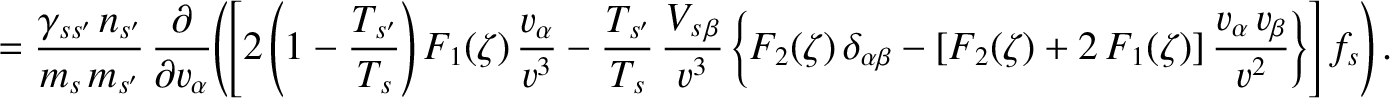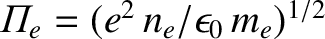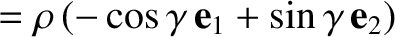(391)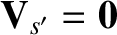(392)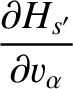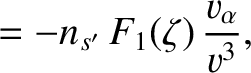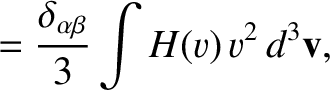(393)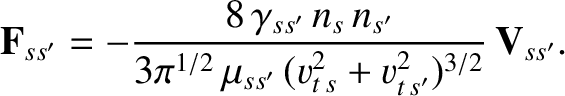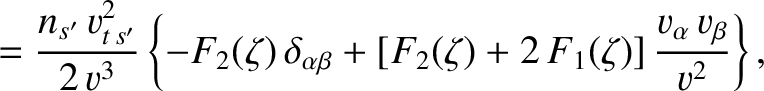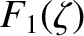and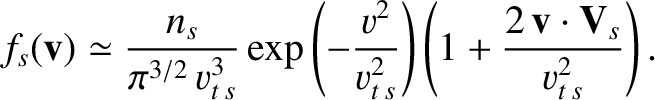(394)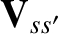(395)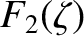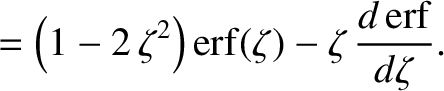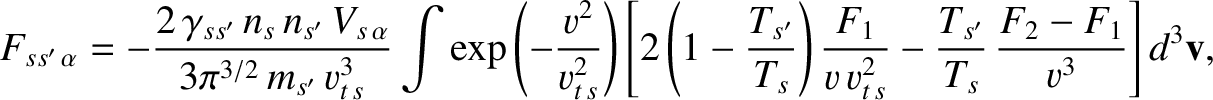(396)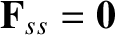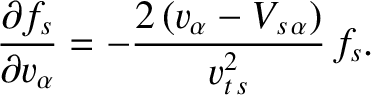In the drift limit, the motions of the electron and ion fluids are sufficiently different that there is little to be gained in rewriting the drift equations in terms of the centre of mass velocity and the plasma current. Instead, let us consider the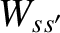components of Eqs. (392) and (396):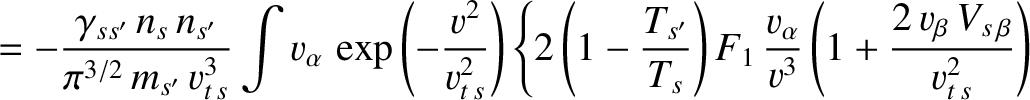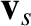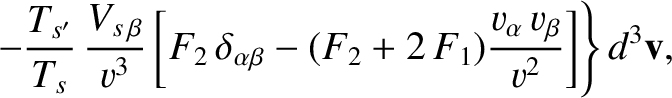(397)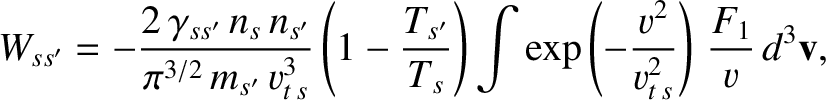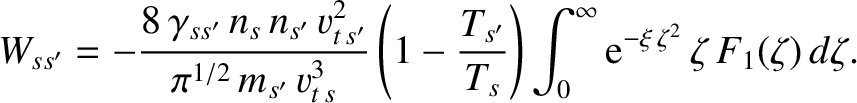(398)

In the above equations, we have neglected all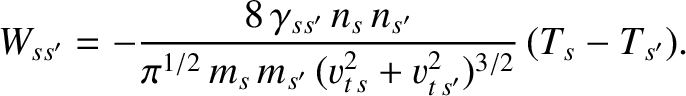terms for the sake of simplicity. Equations (397)-(398) can be inverted to give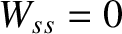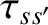(399)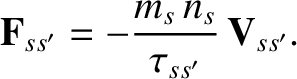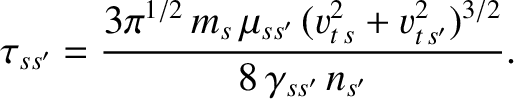(400)

Here,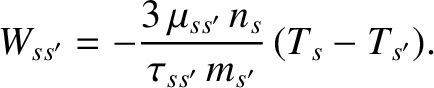is the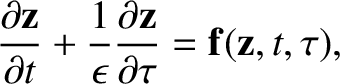velocity, whereas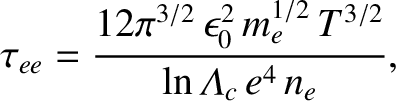(401)

and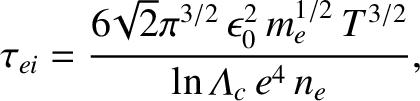(402)

are termed the electron diamagnetic velocity and the ion diamagnetic velocity, respectively.

According to Eqs. (399)-(400), in the drift approximation the velocity of the electron fluid perpendicular to the magnetic field is the sum of thevelocity and the electron diamagnetic velocity. Similarly, for the ion fluid. Note that in the MHD approximation the perpendicular velocities of the two fluids consist of thevelocity alone, and are, therefore, identical to lowest order. The main difference between the two ordering lies in the assumed magnitude of the electric field. In the MHD limit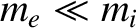(403)

whereas in the drift limit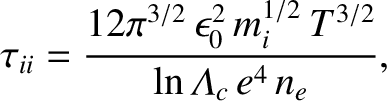(404)

Thus, the MHD ordering can be regarded as a strong (in the sense used in Sect. 2) electric field ordering, whereas the drift ordering corresponds to a weak electric field ordering.

The diamagnetic velocities are so named because the diamagnetic current,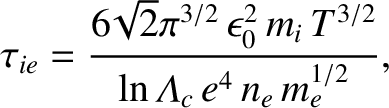(405)

generally acts to reduce the magnitude of the magnetic field inside the plasma.

The electron diamagnetic velocity can be written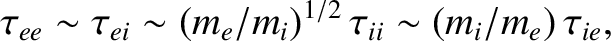(406)

In order to account for this velocity, let us consider a simplified case in which the electron temperature is uniform, there is a uniform density gradient running along the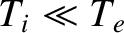-direction, and the magnetic field is parallel to the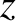-axis--see Fig. 7. The electrons gyrate in the-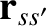plane in circles of radius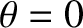. At a given point, coordinate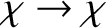, say, on the-axis, the electrons that come from the right and the left have traversed distances of order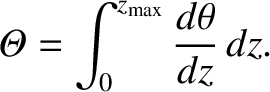. Thus, the electrons from the right originate from regions where the particle density is of order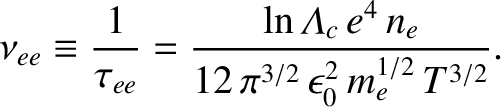greater than the regions from which the electrons from the left originate. It follows that the-directed particle flux is unbalanced, with slightly more particles moving in the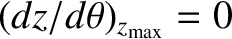-direction than in the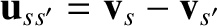-direction. Thus, there is a net particle flux in the-direction: i.e., in the direction of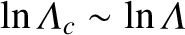. The magnitude of this flux is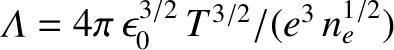(407)

Note that there is no unbalanced particle flux in the-direction, since the-directed fluxes are due to electrons which originate from regions where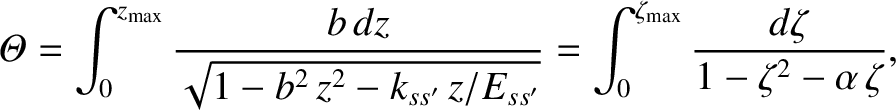. We have now accounted for the first term on the right-hand side of the above equation. We can account for the second term using similar arguments. The ion diamagnetic velocity is similar in magnitude to the electron diamagnetic velocity, but is oppositely directed, since ions gyrate in the opposite direction to electrons.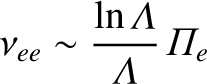The most curious aspect of diamagnetic flows is that they represent fluid flows for which there is no corresponding motion of the particle guiding centres. Nevertheless, the diamagnetic velocities are real fluid velocities, and the associated diamagnetic current is a real current. For instance, the diamagnetic current contributes to force balance inside the plasma, and also gives rise to ohmic heating.Next: Closure in Collisionless Magnetized Up: Plasma Fluid Theory Previous: MHD Equations
Richard Fitzpatrick 2011-03-31